# Signal Separation of Phase-sensitive Optical Time-domain Reflectometry Considering Thermo-mechanical Coupling and 3D Data Matching

Signal Separation of Phase-sensitive Optical Time-domain Reflectometry Considering Thermo-mechanical Coupling and 3D Data Matching
Automation College, Beijing University of Posts and Telecommunications, Beijing 100876, China

Corresponding Author Email:
yzhu@263.net
Page:
65-77
|
DOI:
https://doi.org/10.18280/ts.360109
17 November 2018
|
Accepted:
10 January 2019
|
Published:
30 April 2019
| Citation

OPEN ACCESS

Abstract:

This paper aims to enhance the signal separation accuracy of phase-sensitive optical time-domain reflectometry (Φ-OTDR) under thermo-mechanical coupling (TMC). For this purpose, the Φ-OTDR signal was firstly separated by genetic algorithm-empirical mode decomposition-nonnegative matrix factorization (GA-EMD-NMF). Then, the Kalman-proportional-integral-derivative (PID) method was introduced to further separate the data. Taking the TMC as the input, a feedback model was constructed by the genetic algorithm-radial basis function (GA-RBF) to decouple the TMC. To tackle the 3D Φ-OTDR data, a 3D speeded up robust features (SURF) method was developed to reconstruct the box filter and Gaussian pyramid based on the features of the 3D data, thereby enhancing the positioning accuracy of Φ-OTDR signal. Later, several experiments were carried out in a semi-anechoic room, which proved that the proposed method outperforms the traditional methods. The research findings shed new light on signal separation of Φ-OTDR.

Keywords:

phase-sensitive optical time-domain reflectometry (Φ-OTDR), thermo-mechanical coupling (TMC), 3D data matching

1. Introduction

1.1 Phase-sensitive optical time-domain reflectometry (Φ-OTDR)

Proposed by Taylor and Lee in 1993, Φ-OTDR is a typical monitoring technique for distributed vibrations. Capable of positioning continuous and distributed signals, this technique has been widely applied to health monitoring of large buildings , perimeter security of important places , and so on. However, we hope to grasp its monitoring state more accuracy, understand its vibration mode better and enhance monitoring efficiency. Therefore, it is of great significant for a standard time-domain analytic expression of the Φ-OTDR data. The Φ-OTDR data is a distributed vibration signal. Many scholars have explored the signal extraction and enhanced the signal separation of Φ-OTDR [4, 5], yet failing to consider the impacts of temperature, stress and thermo-mechanical coupling (TMC) on the vibration mode and data purity. The presence of these influencing factors may disturb the monitoring of the target signals and lead to serious positioning errors [6, 7]. These problems must be solved urgently to ensure the effect of Φ-OTDR signal separation.

1.2 TMC

TMC is a common phenomenon in the vibration of new materials (e.g. carbon nanotube, functionally graded materials, magnetorheological elastomers, macro fibre composite and ceramic matrix composite) [8~14], electro-mechanical equipment (e.g. microelectromechanical system oscillators and surface-mounted assembly isolators) [15, 16] and actual facilities (e.g. flexible wicked heat pipes, largescale welded walls and sandwich microplates) [17~19]. Despite the absence of optical fibres-related techniques, all these objects exhibit TMC during the vibration process. This phenomenon also exists in Φ-OTDR applications. However, there is no report on the relationship between TMC and vibration mode.

1.3 Data matching

The data matching methods mainly fall into 1D matching and 2D matching. 1D matching is usually employed to process speech signals. Typical 1D matching approaches include dynamic time warping, hidden Markov method and vector quantization [20~25]. 2D matching mainly targets the grayscale and features of image signals. Popular methods for grayscale matching include mean absolute deviation, sum of absolute differences, sum of squared differences, mean square differences, normalized cross correlation, sequential similarity detection algorithm and sum of absolute transformed difference [26~32], while those for feature matching involves generalized Hough transform, speeded up robust features (SURF), scale invariant feature transform (SIFT) and deep learning [33~37]. In general, the above strategies are applicable to 1D or 2D data matching problems, but not the 3D data of Φ-OTDR (the 3D refers to time, length and TMC).

1.4 Thesis statement

This paper aims to enhance the signal separation accuracy of Φ-OTDR under TMC. For this purpose, the Φ-OTDR signal was firstly separated by genetic algorithm-empirical mode decomposition-nonnegative matrix factorization (GA-EMD-NMF) . Then, the Kalman-proportional-integral-derivative (PID) method was introduced to further separate the data. Taking the TMC as the input, a feedback model was constructed by the genetic algorithm-radial basis function (GA-RBF) to decouple the TMC. To tackle the 3D Φ-OTDR data, a 3D SURF method was developed to reconstruct the box filter and Gaussian pyramid based on the features of the 3D data, thereby enhancing the positioning accuracy of Φ-OTDR signal. Later, several experiments were carried out in a semi-anechoic room, which proved that the proposed method outperforms the traditional methods.

2. Φ-OTDR Signal Seprartion Under TMC

As mentioned before, the data stored in Φ-OTDR contains three types of information: time, length and TMC. The previous research  has shown that signal separation is the first step in the extraction and processing of Φ-OTDR data.

2.1 Signal separation by GA-EMD-NMF

Since the Φ-OTDR data conform to the law of damping vibration and the law of Spalart-Allmaras (SA) turbulence, a damping vibration model was established in the time-TMC plane (Equation (1)) and an SA model was created in the length-TMC plane (Equation (2)) by the EMD method.

${{f}_{1}}(t)={{A}_{1}}{{e}^{-\frac{t}{\tau }}}\sin ({{w}_{1}}t+{{\varphi }_{1}})+{{B}_{1}}$ (1)

${{f}_{2}}(l)={{A}_{2}}\frac{\sin ({{w}_{2}}l+{{\varphi }_{2}})}{{{w}_{2}}l+{{\varphi }_{2}}}+{{B}_{2}}$ (2)

The two models can be superimposed into Equation (3).

$F(t,l)={{f}_{1}}(t)\cdot {{f}_{2}}(l)$ (3)

Following the NMF algorithm, a nonnegative matrix X+ was determined and decomposed into two nonnegative matrices (W+ and H+).

${{X}_{+}}\approx {{W}_{+}}\cdot {{H}_{+}}$ (4)

where, the subscript + is the nonnegative constraint.

To solve Equation (4), the product of matrices W and H can be approached by the original matrix X.

\begin{align} & E(X||WH)=0.5\cdot ||X-W\cdot H||_{F}^{2} \\ & \text{=}0.5\cdot {{\sum\nolimits_{ij}{({{X}_{ij}}-{{W}_{i}}\cdot {{H}_{j}})}}^{2}} \\\end{align} (5)

where, the Euclidean distance is the error between WH and X. This error is minimized when Equation (5) reaches the minimum. The iterative rules of the above equation are as follows:

${{W}_{ik}}\leftarrow {{W}_{ik}}\cdot \frac{X\cdot {{H}^{T}}}{{{W}_{ik}}\cdot H\cdot {{H}^{T}}}$ (6)

${{H}_{ik}}\leftarrow {{H}_{ik}}\cdot \frac{{{W}^{T}}\cdot X}{W\cdot {{W}^{T}}\cdot {{H}_{ik}}}$ (7)

Let d be a low spatial dimension that can roughly describe the original data. Then, d must satisfy (m+n)dmn. The matrices X, W and H are all nonnegative.

Then, the objective function was redefined by the GA as follows:

\begin{align} & {{E}_{1}}({{{\hat{X}}}_{t}}||f(t))=0.5\cdot ||{{X}_{t}}-f(t)||_{F}^{2} \\ & \text{=}0.5\cdot {{\sum\nolimits_{ij}{({{X}_{ij}}-f(t))}}^{2}} \\\end{align} (8)

\begin{align} & {{E}_{2}}({{{\hat{X}}}_{l}}||f(l))=0.5\cdot ||{{X}_{l}}-f(l)||_{F}^{2} \\ & \text{=}0.5\cdot {{\sum\nolimits_{ij}{({{X}_{ij}}-f(l))}}^{2}} \\\end{align}  (9)

$J=0.5{{E}_{1}}+0.5{{E}_{2}}$ (10)

where, J is fitness.

For better convergence, the rotation matrix was defined as a quaternion:

$R=\left[ \begin{matrix} 1-(q_{2}^{2}+q_{3}^{2}) & 2({{q}_{1}}{{q}_{2}}-{{q}_{3}}{{q}_{0}}) & 2({{q}_{1}}{{q}_{3}}+{{q}_{2}}{{q}_{0}}) \\ 2({{q}_{1}}{{q}_{2}}+{{q}_{3}}{{q}_{0}}) & 1-(q_{1}^{2}+q_{3}^{2}) & 2({{q}_{2}}{{q}_{3}}-{{q}_{1}}{{q}_{0}}) \\ 2({{q}_{1}}{{q}_{3}}-{{q}_{2}}{{q}_{0}}) & 2({{q}_{2}}{{q}_{3}}+{{q}_{1}}{{q}_{0}}) & 1-(q_{1}^{2}+q_{2}^{2}) \\\end{matrix} \right]$ (11)

The above equation can be rewritten as:

$\hat{X}={{\left[ \begin{matrix} {{f}_{\infty }}(t) \\ {{f}_{\infty }}(l) \\ {{F}_{\infty }}(t,l) \\ 1 \\\end{matrix} \right]}^{\text{T}}}={{\left[ \begin{matrix} f(t) \\ f(l) \\ F(t,l) \\ 1 \\\end{matrix} \right]}^{\text{T}}}\cdot \left[ \begin{matrix} {} & {} & {} & \Delta f(t) \\ {} & R & {} & \Delta f(l) \\ {} & {} & {} & \Delta F(t,l) \\ 0 & 0 & 0 & 1 \\\end{matrix} \right]$ (12)

Note that the variation convergence steps of △f(t), △f(l) and △F(t,l) are 0.01, the convergence steps of q0, q1, q2 and q3 are 0.1, and Q=q0+q1·i+q2·j+q3·k.

2.2 Signal separation by Kalman PID

Considering the high noise level of Φ-OTDR data, Kalman PID was introduced to further separate the data. The original signal of Φ-OTDR can be expressed as a matrix of m rows (time i) and n column (length j). Φ-OTDR vibration signal are about displacement. The velocity and acceleration can be obtained by subtracting the data from the adjacent position on the timeline:

\begin{align} & M{{I}_{ij}}=dat{{a}_{ij}}i=1,...,mj=1,...,n \\ & M{{P}_{ij}}=M{{I}_{(i+1)j}}-M{{I}_{ij}}i=1,...,m-1j=1,...,n \\ & M{{D}_{ij}}=M{{I}_{(i+1)j}}-M{{I}_{ij}}i=1,...,m-2j=1,...,n \\\end{align} (13)

where, dataij is the original data; MIij is TMC; MPij is velocity; MDij is acceleration. The above equation can be extended as follows:

\begin{align} & M{{I}_{ij}}\to GAEMDNMF\to {{f}_{1}}(t)\And {{f}_{2I}}(l) \\ & M{{P}_{ij}}\to GAEMDNMF\to {{f}_{1}}^{\prime }(t)\And {{f}_{2P}}(l) \\ & M{{D}_{ij}}\to GAEMDNMF\to {{f}_{1}}^{\prime \prime }(t)\And {{f}_{2D}}(l) \\\end{align} (14)

These three length functions cannot directly derive the results from the mean number or the median number. Thus, the two numbers were obtained by the following equation.

\left\{ \begin{align} & {{f}_{2mean}}(l)=[{{f}_{2P}}(l)+{{f}_{2I}}(l)+{{f}_{2D}}(l)]/3 \\ & {{f}_{2middle}}(l)=middle[{{f}_{2P}}(l),{{f}_{2I}}(l),{{f}_{2D}}(l)] \\\end{align} \right. (15)

Then, the Kalman filter was introduced to process the results. The five classical equations of the filter are listed below.

(1) State prediction equation:

$\eta (k|k-1)=\Psi \cdot \eta (k-1|k-1)+\Upsilon \cdot U(k)$ (16)

(2) Covariance prediction equation:

$P(k|k-1)=\Psi \cdot \eta (k|k-1)\cdot {{\Psi }^{\text{T}}}+Q$ (17)

(3) Covariance estimation equation:

$\eta (k|k)=\eta (k|k-1)+Kg(k)\cdot (\Gamma (k)-H\cdot \eta (k|k-1))$ (18)

(4) Kalman gain matrix equation:

$Kg(k)=P(k|k-1)\cdot H\cdot {{[H\cdot P(k|k-1)\cdot {{H}^{\text{T}}}\text{+R}]}^{-1}}$ (19)

(5) State estimation equation:

$P(k|k)=(E-kg(k)\cdot H)\cdot P(k|k-1)$ (20)

where, k is the number of frames of successive images. Through Kalman filtering, the positions of the previous and next frames can be obtained, laying the basis for vibration convergence.

According to Equation (18), the covariance matrices can be obtained for the time, length and TMC curves. Then, these matrices can be co-calculated by the following equation:

$\eta =\min ({{\eta }_{P}},{{\eta }_{I}},{{\eta }_{D}})$ (21)

2.3 Feedback model

The Φ-OTDR vibration signals are affected by the temperature and stress on the fibre. Since the Φ-OTDR device must use a single mode fibre, it is improper to decompose the signals by Brillouin optical time-domain reflectometer (BOTDR), a popular fibre optic strain and temperature distributed sensing system. Here, Brillouin central frequency shift is combined with Rayleigh frequency shift to separate the information of temperature and strain :

\left\{ \begin{align} & \Delta {{f}_{Brillouin-center}}={{k}_{1}}\cdot \Delta T+{{k}_{2}}\cdot \Delta \varepsilon \\ & \Delta {{f}_{Rayleigh}}={{k}_{3}}\cdot \Delta T+{{k}_{4}}\cdot \Delta \varepsilon \\\end{align} \right.  (22)

where, k1, k2, k3 and k4 were defined in advance.

For the given temperature and strain, a series of feedback models can be established :

\begin{align} & {{\Gamma }_{1}}(\Delta T,\Delta \varepsilon )=\left[ \begin{matrix} {{K}_{1}} & {{K}_{2}} \\\end{matrix} \right]\cdot {{\left[ \begin{matrix} \Delta T & \Delta \varepsilon \\\end{matrix} \right]}^{\text{T}}} \\ & {{\Gamma }_{2}}(\Delta T,\Delta \varepsilon )=\left[ \begin{matrix} {{K}_{1}} & {{K}_{2}} \\\end{matrix} \right]\cdot {{\left[ \begin{matrix} {{e}^{\Delta T}} & {{e}^{\Delta \varepsilon }} \\\end{matrix} \right]}^{\text{T}}} \\ & {{\Gamma }_{3}}(\Delta T,\Delta \varepsilon )=\left[ \begin{matrix} {{K}_{1}} & {{K}_{2}} \\\end{matrix} \right]\cdot {{\left[ \begin{matrix} {{e}^{-\Delta T}} & {{e}^{-\Delta \varepsilon }} \\\end{matrix} \right]}^{\text{T}}} \\ & {{\Gamma }_{4}}(\Delta T,\Delta \varepsilon )=\left[ \begin{matrix} {{K}_{1}} & {{K}_{2}} \\\end{matrix} \right]\cdot {{\left[ \begin{matrix} \Delta {{T}^{-1}} & \Delta {{\varepsilon }^{-1}} \\\end{matrix} \right]}^{\text{T}}} \\ & {{\Gamma }_{5}}(\Delta T,\Delta \varepsilon )=\left[ \begin{matrix} {{K}_{1}} & {{K}_{2}} \\\end{matrix} \right]\cdot {{\left[ \begin{matrix} \Delta {{T}^{2}} & \Delta {{\varepsilon }^{2}} \\\end{matrix} \right]}^{\text{T}}} \\ & {{\Gamma }_{6}}(\Delta T,\Delta \varepsilon )=\left[ \begin{matrix} {{K}_{1}} & {{K}_{2}} \\\end{matrix} \right]\cdot {{\left[ \begin{matrix} \Delta {{T}^{-2}} & \Delta {{\varepsilon }^{-2}} \\\end{matrix} \right]}^{\text{T}}} \\\end{align} (23)

where, K1 and K2 are nonnegative diagonal matrices:

\left\{ \begin{align} & {{K}_{1}}\text{=diag }\!\![\!\!\text{ }\Delta {{T}^{2}}\text{ }\!\!]\!\!\text{ } \\ & {{K}_{2}}\text{=diag }\!\![\!\!\text{ }\Delta {{\varepsilon }^{2}}\text{ }\!\!]\!\!\text{ } \\ \end{align} \right. (24)

The other form of the feedback device can be obtained by the state equation:

$(\Delta \dot{T},\Delta \dot{\varepsilon })={{\Gamma }_{4}}(\Delta T,\Delta \varepsilon )$ (25)

The stability of the feedback device can be proved by the Lyapunov-based decision equation :

$V(\Delta T,\Delta \varepsilon )={{\left[ \begin{matrix} \Delta T \\ \Delta \varepsilon \\\end{matrix} \right]}^{\text{T}}}\cdot P\cdot \left[ \begin{matrix} \Delta T \\ \Delta \varepsilon \\\end{matrix} \right]=\Delta {{T}^{2}}+\Delta {{\varepsilon }^{2}}$ (26)

where, P=I such that the former is a positive definite matrix. Taking the derivative of Equation (25), we have:

\begin{align} & \frac{d}{dt}{{V}_{1}}(\Delta T,\Delta \varepsilon )=2\left[ \begin{matrix} \Delta T & \Delta \varepsilon \\\end{matrix} \right]{{\left[ \begin{matrix} \Delta \dot{T} & \Delta \dot{\varepsilon } \\\end{matrix} \right]}^{\text{T}}} \\ & =2\left[ \begin{matrix} \Delta T & \Delta \varepsilon \\\end{matrix} \right]\left[ \begin{matrix} {{K}_{1}} & {{K}_{2}} \\\end{matrix} \right]\cdot {{\left[ \begin{matrix} \Delta T & \Delta \varepsilon \\\end{matrix} \right]}^{\text{T}}} \\ & \frac{d}{dt}{{V}_{2}}(\Delta T,\Delta \varepsilon )=2\left[ \begin{matrix} \Delta T & \Delta \varepsilon \\\end{matrix} \right]{{\left[ \begin{matrix} \Delta \dot{T} & \Delta \dot{\varepsilon } \\\end{matrix} \right]}^{\text{T}}} \\ & =2\left[ \begin{matrix} \Delta T & \Delta \varepsilon \\\end{matrix} \right]\left[ \begin{matrix} {{K}_{1}} & {{K}_{2}} \\\end{matrix} \right]\cdot {{\left[ \begin{matrix} {{e}^{\Delta T}} & {{e}^{\Delta \varepsilon }} \\\end{matrix} \right]}^{\text{T}}} \\ & \frac{d}{dt}{{V}_{3}}(\Delta T,\Delta \varepsilon )=2\left[ \begin{matrix} \Delta T & \Delta \varepsilon \\\end{matrix} \right]{{\left[ \begin{matrix} \Delta \dot{T} & \Delta \dot{\varepsilon } \\\end{matrix} \right]}^{\text{T}}} \\ & =2\left[ \begin{matrix} \Delta T & \Delta \varepsilon \\\end{matrix} \right]\left[ \begin{matrix} {{K}_{1}} & {{K}_{2}} \\\end{matrix} \right]\cdot {{\left[ \begin{matrix} {{e}^{-\Delta T}} & {{e}^{-\Delta \varepsilon }} \\\end{matrix} \right]}^{\text{T}}} \\ & \frac{d}{dt}{{V}_{4}}(\Delta T,\Delta \varepsilon )=2\left[ \begin{matrix} \Delta T & \Delta \varepsilon \\\end{matrix} \right]{{\left[ \begin{matrix} \Delta \dot{T} & \Delta \dot{\varepsilon } \\\end{matrix} \right]}^{\text{T}}} \\ & =2\left[ \begin{matrix} \Delta T & \Delta \varepsilon \\\end{matrix} \right]\left[ \begin{matrix} {{K}_{1}} & {{K}_{2}} \\\end{matrix} \right]\cdot {{\left[ \begin{matrix} \Delta {{T}^{-1}} & \Delta {{\varepsilon }^{-1}} \\\end{matrix} \right]}^{\text{T}}} \\ & \frac{d}{dt}{{V}_{5}}(\Delta T,\Delta \varepsilon )=2\left[ \begin{matrix} \Delta T & \Delta \varepsilon \\\end{matrix} \right]{{\left[ \begin{matrix} \Delta \dot{T} & \Delta \dot{\varepsilon } \\\end{matrix} \right]}^{\text{T}}} \\ & =2\left[ \begin{matrix} \Delta T & \Delta \varepsilon \\\end{matrix} \right]\left[ \begin{matrix} {{K}_{1}} & {{K}_{2}} \\\end{matrix} \right]\cdot {{\left[ \begin{matrix} \Delta {{T}^{2}} & \Delta {{\varepsilon }^{2}} \\\end{matrix} \right]}^{\text{T}}} \\ & \frac{d}{dt}{{V}_{6}}(\Delta T,\Delta \varepsilon )=2\left[ \begin{matrix} \Delta T & \Delta \varepsilon \\\end{matrix} \right]{{\left[ \begin{matrix} \Delta \dot{T} & \Delta \dot{\varepsilon } \\\end{matrix} \right]}^{\text{T}}} \\ & =2\left[ \begin{matrix} \Delta T & \Delta \varepsilon \\\end{matrix} \right]\left[ \begin{matrix} {{K}_{1}} & {{K}_{2}} \\\end{matrix} \right]\cdot {{\left[ \begin{matrix} \Delta {{T}^{-2}} & \Delta {{\varepsilon }^{-2}} \\\end{matrix} \right]}^{\text{T}}} \\\end{align}  (27)

Hence, $d/dt V_{1,2,3,4,5,6} (∆T,∆ε)≤0$, revealing that the feedback devices are all stable.

The feedback effect differs from device to device. Besides, the same device may output different feedbacks in the decompositions of P, I and D. Then, a 6×3 weight matrix was constructed, and the objective function was set up as follows :

$J=\left\| \left[ \begin{matrix} {{f}_{2P}}(l) \\ {{f}_{2I}}(l) \\ {{f}_{2D}}(l) \\\end{matrix} \right]-{{f}_{2kalman}}(l)\left[ \begin{matrix} 1 \\ 1 \\ 1 \\\end{matrix} \right] \right\|$ (28)

The weight matrix was traversed or optimized to satisfy the minimum condition of the objective function.

2.4 TMC

TMC is an objective phenomenon arising from the temperature-induced stress changes. In Φ-OTDR, the effect of this phenomenon on vibration cannot be identified accurately with the existing methods. Therefore, the RBF network was adopted to overcome the problem.

As a feedforward network, the RBF network usually consists of three layers. The first layer is an input layer containing the source nodes. The number of these nodes is determined by the dimension of the input signal. The second layer is a hidden layer, whose number of nodes depends on the specific problem. The transformation function of hidden layer nodes is a nonnegative and nonlinear RBF symmetric and attenuated for the centre point. The data of the hidden layer are a set of RBFs derived from the input data, each of which can be solved by the Gaussian basis function. The third layer is an output layer that responds to the input layer mode. The data output of the hidden and output layers both follow the principle of weighted superposition. The transformation is linear from the hidden layer to the output layer, but that from the input layer to the hidden layer is nonlinear. The topology of the RBF network is illustrated in Figure 1.

## 1.png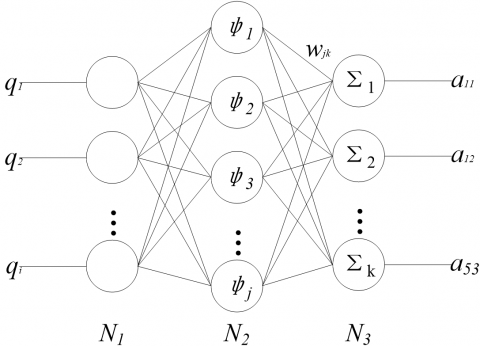Figure 1. Topology of the RBF network

In this paper, the RBF network is designed as a regularization network. The number of input, hidden and output layer nodes was respectively set as N1=2, N2=2 and N3=n with n being the number of points on the time axis of the Φ-OTDR data. Let [q1, q2]T be the input of the network. Then, the output of the j-th hidden layer node can be expressed as:

$\varphi (r)=\exp \left\{ -\frac{{{r}^{2}}}{2{{\sigma }^{2}}} \right\},r={{\left\| {{q}_{i}}-\tilde{q} \right\|}^{2}}$ (29)

where, $q ̃$ is the sample data centre, a 2D vector of the Gaussian basis function; σ is the sample variance of the Gaussian basis function. Following the linear output principle, the output layer data can be obtained by the linear combination from the output to the hidden layer.

The convergence of the RBF network can be described as:

$\delta \text{=}\frac{{{r}_{\max }}}{\sqrt{2P}}$ (30)

where, P is the number of samples.

According to the least mean squares algorithm, the weight of the formula can be adjusted as:

$\Delta {{w}_{jk}}\text{=}\eta ({{r}_{\max }}-W\Phi ){{\varphi }_{j}}$ (31)

where, W is the weight matrix from hidden layer to output layer; η is the learning speed; φ is a vector of hidden nodes.

The output of each output layer node can be determined as:

${{a}_{k}}=\sum\limits_{j=1}^{J}{{{w}_{jk}}\cdot {{\varphi }_{j}}}$ (32)

where, wjk are the connection weights between hidden and output layer nodes, i.e. an N2×N3 matrix.

Next, the GA was selected to calculate the weight matrix from the hidden layer to the output layer . Inspired by natural selection and genetic mechanism, the GA can converge to the global optimal solution by fitness function with the probability of 1, without requiring derivative or other information. Based on the data samples in the RBF network, the said weight matrix can be calculated in the following steps:

(1) Generate a set of random weights from the hidden layer to the output layer, treat each row in the N2×N3 matrix as a population, and regard each element as an independent entity in the population.

(2) Encode each element with a number between 0 and 1 with the step size of 0.1 and ensure that the sum of n elements in each line is 1.

(3) Establish the fitness function according to the sample input:

$f=\left\| Q\cdot W-\bar{A} \right\|$ (33)

where, f  is fitness; Q is the input vector; is the weight matrix; $A ̅$  is the expected output.

Perform continuous iterations and mutations according to the fitness function. Adopt the result of genetic coding when the fitness $A ̅$ is reduced; otherwise, change that result.

3. Signal Matchingunder Data Deviation

In Φ-OTDR, the TMC varies with the Rayleigh scattering of the light in optical fibre. The variation is intensified by the high level of noises. Even the same vibration source may generate different signals, owing to the difference in noise, time, length and TMC. In view of these, this section screens the errors in Φ-OTDR data through fuzzy c-means (FCM) clustering before matching the data with 3D SURF.

3.1 Signal matching based on 3D SURF

Compared with the SIFT, the 3D SURF is a simple and fast algorithm to extract interest points and describe eigenvectors. The traditional SURF method contains five steps, namely, constructing Hessian matrix, calculating eigenvalue, constructing Gaussian pyramid, determining the principal direction of feature point, locating feature points and constructing feature descriptors. However, the traditional method only applies to 2D data like images. In 3D SURF, the applicable scope is extended to 3D data. The specific steps of 3D SURF are introduced below.

First, the Hessian matrix was built as a 3D model with time, length and TMC as the x, y and z axes, respectively:

$H(f(x,y,z))=\left[ \left. \begin{matrix} \frac{{{\partial }^{3}}f}{\partial {{x}^{3}}} & \frac{{{\partial }^{3}}f}{\partial {{x}^{2}}\partial y} & \frac{{{\partial }^{3}}f}{\partial {{x}^{2}}\partial z} \\ \frac{{{\partial }^{3}}f}{\partial {{x}^{2}}\partial y} & \frac{{{\partial }^{3}}f}{\partial x\partial {{y}^{2}}} & \frac{{{\partial }^{3}}f}{\partial x\partial y\partial z} \\ \frac{{{\partial }^{3}}f}{\partial {{x}^{2}}\partial z} & \frac{{{\partial }^{3}}f}{\partial x\partial y\partial z} & \frac{{{\partial }^{3}}f}{\partial x\partial {{z}^{2}}} \\\end{matrix} \right|\left. \begin{matrix} \frac{{{\partial }^{3}}f}{\partial {{x}^{2}}\partial y} & \frac{{{\partial }^{3}}f}{\partial x\partial {{y}^{2}}} & \frac{{{\partial }^{3}}f}{\partial x\partial y\partial z} \\ \frac{{{\partial }^{3}}f}{\partial x\partial {{y}^{2}}} & \frac{{{\partial }^{3}}f}{\partial {{y}^{3}}} & \frac{{{\partial }^{3}}f}{\partial {{y}^{2}}\partial z} \\ \frac{{{\partial }^{3}}f}{\partial x\partial y\partial z} & \frac{{{\partial }^{3}}f}{\partial {{y}^{2}}\partial z} & \frac{{{\partial }^{3}}f}{\partial y\partial {{z}^{2}}} \\\end{matrix} \right|\begin{matrix} \frac{{{\partial }^{3}}f}{\partial {{x}^{2}}\partial z} & \frac{{{\partial }^{3}}f}{\partial x\partial y\partial z} & \frac{{{\partial }^{3}}f}{\partial x\partial {{z}^{2}}} \\ \frac{{{\partial }^{3}}f}{\partial x\partial y\partial z} & \frac{{{\partial }^{3}}f}{\partial {{y}^{2}}\partial z} & \frac{{{\partial }^{3}}f}{\partial y\partial {{z}^{2}}} \\ \frac{{{\partial }^{3}}f}{\partial x\partial {{z}^{2}}} & \frac{{{\partial }^{3}}f}{\partial y\partial {{z}^{2}}} & \frac{{{\partial }^{3}}f}{\partial {{z}^{3}}} \\\end{matrix} \right]$ (34)

Then, the Hessian matrix was filtered by Gaussian function:

\begin{align} & H(x,{{\sigma }_{1}},{{\sigma }_{2}},{{\sigma }_{3}})= \\ & \left[ \left. \begin{matrix} {{L}_{xxx}} & {{L}_{xxy}} & {{L}_{xxz}} \\ {{L}_{xxy}} & {{L}_{xyy}} & {{L}_{xyz}} \\ {{L}_{xxz}} & {{L}_{xyz}} & {{L}_{xzz}} \\\end{matrix} \right|\left. \begin{matrix} {{L}_{xxy}} & {{L}_{xyy}} & {{L}_{xyz}} \\ {{L}_{xyy}} & {{L}_{yyy}} & {{L}_{yyz}} \\ {{L}_{xyz}} & {{L}_{yyz}} & {{L}_{yzz}} \\\end{matrix} \right| \right.\left. \begin{matrix} {{L}_{xxz}} & {{L}_{xyz}} & {{L}_{xzz}} \\ {{L}_{xyz}} & {{L}_{yyz}} & {{L}_{yzz}} \\ {{L}_{xzz}} & {{L}_{yzz}} & {{L}_{zzz}} \\\end{matrix} \right] \\\end{align} (35)

After that, the discriminant of the Hessian matrix was calculated as follows:

$\det (H)=\sum\limits_{i,j,k=1}^{3}{[\left| H(i,:,:) \right|+\left| H(:,j,:) \right|+\left| H(:,:,k) \right|]}$ (36)

The discriminant reflects the local maximum value, and determines if the current point is brighter or darker than the adjacent points, laying the basis for eigenvalue positioning. The derivation formulas of the discrete data can be expressed as:

\begin{align} & Dx(x,y,z)=f(x+1,y,z)-f(x,y,z) \\ & Dxx(x,y,z)=Dx(x+1,y,z)-Dx(x,y,z) \\ & Dxxx(x,y,z)=Dxx(x+1,y,z)-Dxx(x,y,z) \\\end{align} (37)

where, the first derivative is the TMC difference between the two adjacent points; the second derivative is the difference between the two adjacent points of the first derivative; the third derivative is the difference between the two adjacent points of the second derivative.

## 2.jpg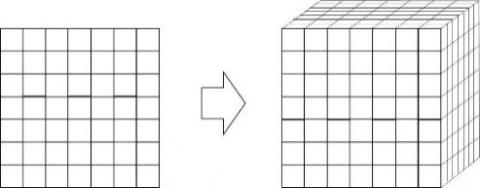Figure 2. A typical 3D box filter

In the traditional SURF, the Gaussian pyramid is constructed on a template using different box filters. For 3D SURF, the box filters were extended from 2D to 3D (Figure 2). The box filters are of different scales, ranging from 5×5×5 to 21×21×21. Together, these box filters form a 3D Gaussian pyramid (Figure 3).

## 3.jpg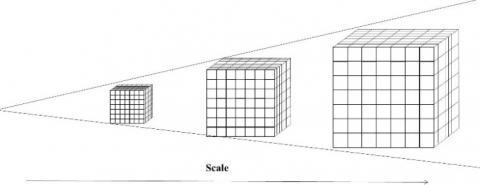Figure 3. 3D Gaussian pyramid

All data points were processed through the Hessian matrix and Gaussian pyramid. Each data point was compared with 26 points in the neighborhood of the scale space in Figure 4, where the feature points are shown in green. To identify the stable feature points, the feature points with weak energy and location error were filtered out by a pre-set threshold.

## 4.jpg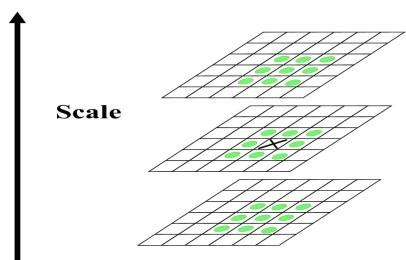Figure 4. Feature points

Figure 5 shows the Haar-like features in the spherical neighborhood of a feature point. In the 3D neighborhood, the Haar-like features of all the feature points within the 60° pyramid were counted. Then, the neighborhood was scanned with 0.2 radians. The conical direction with the maximum value is the main direction of the feature point.

## 5.jpgFigure 5. Principal direction

A 4×4×4 area around the feature point was selected in the 3D SURF algorithm, with the principal direction of the feature point as the direction of this area. Six Haar features of the 25 data points were counted in each sub-area:

\begin{align} & featur{{e}_{No.1}}\to \sum{dx},featur{{e}_{No.2}}\to \sum{\left| dx \right|} \\ & featur{{e}_{No.3}}\to \sum{dy},featur{{e}_{No.4}}\to \sum{\left| dy \right|} \\ & featur{{e}_{No.5}}\to \sum{dz},featur{{e}_{No.6}}\to \sum{\left| dz \right|} \\\end{align} (38)

Therefore, there were 4×4×4×6=384 descriptors. The Euclidean distance between two feature points was regarded as the matching degree. The Hessian discriminants of both points must be positive or negative at the same time.

3.2 FCM screening of matching points

In traditional SURF, the matching points are mainly screened by thresholding and detection of sampling consistency. The screening effect can be further improved by unsupervised clustering in machine learning. To separate correct and wrong data, unsupervised clustering first allocates datasets into different classes by similarity. The most popular clustering methods are k-means clustering, hierarchical clustering, self-organizing map and FCM clustering.

Among them, k-means and FCM boast relatively short runtime and high accuracy. However, k-means has unstable and random initial points, which may destabilize the clustering results, while FCM is prone to local optimum trap due to the manual determination of the number of clusters. The FCM was adopted for the screening of matching points in 3D SURF, because the only object is to separate correct clusters from wrong clusters. The specific steps of FCM are introduced as follows:

(1) Standardize the data matrix with the Euclidean distance between the descriptors of two matching points as the source data;

(2) Establish the fuzzy similarity matrix and initialize the membership matrix;

(3) Start the iteration until the target function converges to the minimum;

(4) Determine the class of the data by the final membership matrix according to the iterative results.

4. Experiments

## 6.jpg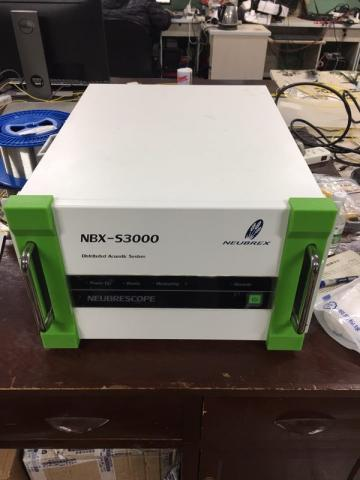Figure 6. Φ-OTDR instrument

In our experiments, the Φ-OTDR instrument is an NBX-S3000 device manufactured by the Japanese company Nebrex (Figure 6). The parameters of the instrument are as follows: sampling rate=4,000Hz, recording time=10s, recording distance=100m and spatial resolution=0.1m.

The experiments were carried out in an anechoic room to minimize the environmental noises and ensure the vibration effect. The environment is shown in Figures 7 and 8. Note that the fibre in Figure 7 clings to the ground, and the instrument in Figure 8 is placed on a damping table.

## 7.jpgFigure 7. Layout of optical fibre

## 8.jpgFigure 8. Anechoic room

During the experiments, the temperature and strain were adjusted manually. Specifically, the optical fibre was placed in a thermostat to regulate the temperature and the strain was controlled by changing the tension on the fibre. The fibre temperature and strain were measured by the BOTDR.

4.1 Precepted distance on the length axis at different temperatures and strains

The signals of Φ-OTDR vary with time and length. Figure 9 presents the effect of single point knocking on the optical fibre. It can be seen that the peak vibration occurred at the vertical intersection point between the vibration source and the optical fibre. Besides, the signal gradually weakened from the intersection to each end and eventually disappeared at the end.

Figure 10 provides the waterfall plots of four different Φ-OTDR signals emitted from the same vibration source at different conditions. Note that the x axis is length and the y axis is time. As shown in the figure, the band width and brightness are positively correlated with the strain and negatively with the environmental temperature.

## 9.jpg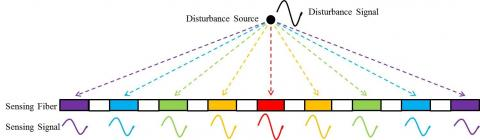Figure 9. Perception model in length axis

## 10.png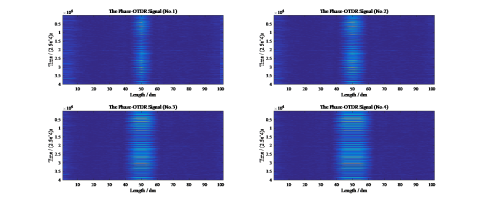Figure 10. Waterfall plots of four Φ-OTDR signals

The ranges of the Φ-OTDR signals were collected at different temperatures and strains. The data points and their residuals are recorded in Figure 11. Discrete data points were fitted into a curve. The value on the curve was the expected output.

## 11.png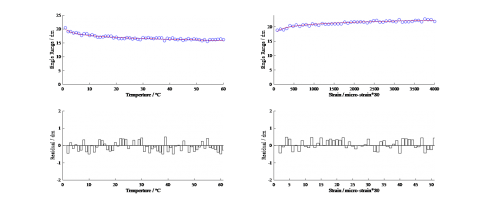Figure 11. Data points and their residuals at different temperatures and strains

As shown in Figure 11, the signal range is positively correlated with the strain and negatively with the temperature, and the data points basically obeyed the exponential distribution. Moreover, the residuals between the fitted curves and the actual data fluctuated around the zero point, indicating that these curves are effective and in need of further improvement.

Next, 1,000 groups of experiments were conducted with another vibration source to verify the above result. The experimental results are shown in Figure 12, where effective points are in black and invalid ones are in white. It can be seen that the RBF method achieved an accuracy as high as 99.233%.

## 12.png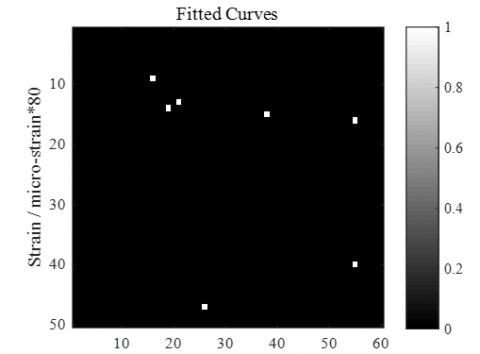Figure 12. Results of validation experiments

4.2 Precepted distance on the time axis at different temperatures and strains

A standard 5-hammer vibration device was taken as the vibration source (Figures 13 and 14). The five percussion hammers were equally distributed on the knocker. The distance between two strikes was 10cm. As shown in Figure 15, the five vibration signals were not the same on the time axis and should be separated.

## 13.png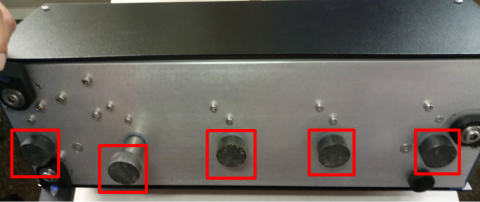Figure 13. 5 percussion hammers

## 14.jpg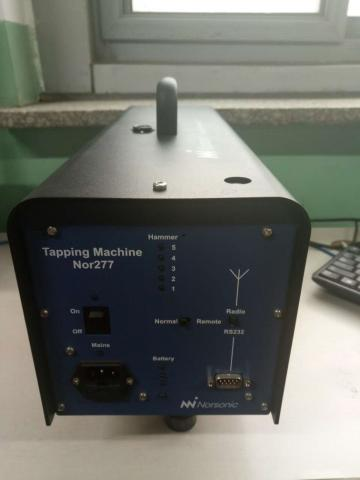Figure 14. Vibration source

## 15.jpg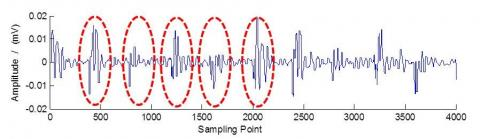Figure 15. Vibration signals of the five hammers

## 16.png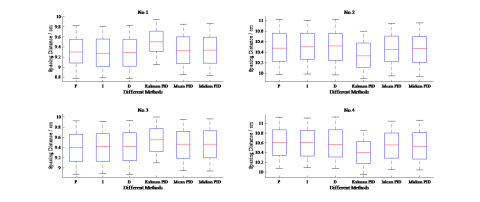Figure 16. Experimental results of the six signal separation methods

The four gaps, each of which is 10cm, between the five striking points were solved by the following six signal separation methods: the proportional (P) method, the integral (I) method, the derivative (D) method, the Kalman PID, the mean PID and the median PID. As suggested by the name, the mean PID refers to the mean value of the P, I and D and the median PID refers to the median value of the P, I and D. Each of the six methods was trained 1,000 times in four spaces and subjected to 1,000 groups of experiments. The experimental results are displayed in Figure 16.

As shown in Figure 16, the P, I and D methods achieved similar positioning effects, with the mean error and error range falling in 0.5~0.7cm and -0.55~0.55cm, respectively. The mean PID and median PID slightly outperformed the P, I and D methods, with the mean error and error range falling in 0.5~0.65cm and -0.52~0.52cm, respectively. The Kalman PID boasted the best positioning effect, with the mean error and error range falling in 0.35~0.45cm and -0.45~0.45cm, respectively. Overall, the Kalman PID improved the positioning accuracy by 1% and reduced the error range by 10% in the four spaces. This is because the Kalman PID adopts the minimum variance matrix of the P, I and D methods and the step-wise filtering.

4.2.2 Advantage of combined feedback model

The six feedback models in Equation (23) were trained 1,000 times in the four spaces and subjected to 1,000 groups of experiments, with Kalman PID as the signal separation method. The experimental results of these models are shown in Figure 17.

## 17-1.png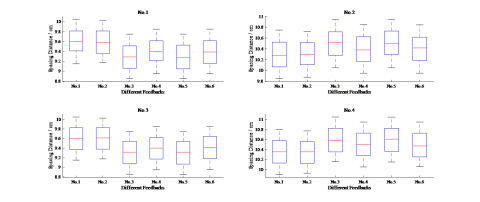## 17-2.pngFigure 17. Experimental results of different feedback models

As can be seen from Figure 17, the first and second feedback models boasted the most accurate positioning effects among the six models, and their mean errors were between 0.35cm and 0.4cm. The six feedback models had similar error ranges.

## 18-1.png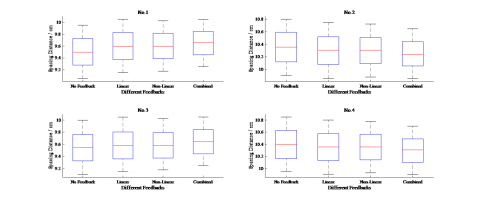## 18-2.pngFigure 18. Experimental results of the combined feedback model

For better accuracy, the first feedback model (linear) and second feedback model (nonlinear) were combined into a model that considers both linear and nonlinear features. Then, the combined model was applied to 1,000 groups of experiments, with Kalman PID as the signal separation method. The experimental results of the combined model are compared with those of the first feedback model, the second feedback model and the no feedback scenario (Figure 18). It is clear that the combined feedback model outshined both linear and nonlinear models, with the mean error and error range falling in 0.2~0.4cm and -0.4~0.4cm, respectively.

4.2.3 Advantage of the proposed TMC method

In Φ-OTDR technology, the effects of temperature and strain are much more complex than what is described by linear and nonlinear feedback models. In the 1,000 groups of experiments, the expected output was set to 10cm, 10cm, 10cm and 10cm. Then, 700 groups of experiments were randomly selected as training samples and the remaining 300 groups of experiments were adopted as testing samples. The RBF method and the GA-RBF method were trained by the training samples. The results of the testing samples of combined feedback model, RBF and GA-RBF are shown in Figure 19.

## 19.png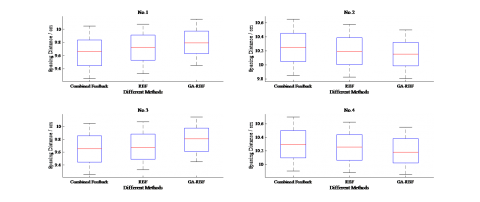Figure 19. Experimental results of TMC methods

It can be seen from Figure 19 that both the RBF and GA-RBF had better results than the combined feedback model. In addition, the GA-RBF, with a mean error of 0.2cm and error range of -0.3~0.3cm, outperformed than the RBF. These results show that RBF and GA-RBF are both useful TMC methods.

To further verify the effect of these algorithms, several experiments were carried out using these TMC methods under 60 different temperatures and 50 different strains. The experimental results are presented in Figure 20, where the effective points are in black and the invalid ones are in white.

## 20.png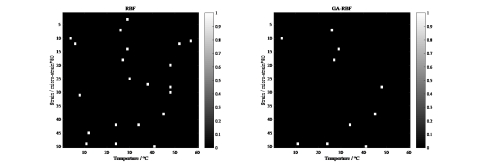Figure 20. Experimental results of different TMC methods

As shown in Figure 20, the effective rate of RBF was 99.233% and that of GA-RBF was 99.7%. This means GA has effectively improved the performance of RBF.

4.3 Results of 3D SURF

Based on Kalman PID and the proposed TMC, the positioning accuracy should be further enhanced by signal matching. Here, 1,000 groups of experiments are conducted by the traditional SURF, 3D SURF and no matching method. The experimental results are shown in Figure 21.

As shown in Figure 21, 3D SURF and SURF both outperformed the no matching method. Besides, 3D SURF, with mean error and error range of 0.1cm and -0.25~0.25cm, respectively, achieved better results than SURF. Hence, both 3D SURF and SURF are desirable feature matching approaches.

To further verify the effect of these algorithms, several experiments were carried out using these TMC methods under 60 different temperatures and 50 different strains. The experimental results are presented in Figure 22, where the effective points are in black and the invalid ones are in white.

## 21.pngFigure 21. Experimental results of different data matching methods

## 22.pngFigure 22. Experimental results of different data matching methods

As shown in Figure 22, the effective rate of SURF was 99% and that of 3D SURF was 99.633%. Obviously, 3D SURF enjoys better results than the traditional SURF.

5. Conclusion

This paper proposes a novel signal separation method for Φ-OTDR to enhance the positioning accuracy. By this method, the signals were divided into P, I and D, and screened by Kalman filter, respectively. The Kalman PID can achieve good separation effect and positioning accuracy. During signal separation, the TMC was considered and solved by different feedback models, combined feedback model and GA-RBF. Meanwhile, the data deviation was also considered and solved. To process the 3D information, 3D SURF was developed from the traditional SURF. Through a number of experiments, it is proved that the Kalman PID separation method, GA-RBF TMC method and 3D SURF data matching method can improve the signal separation and enhance positioning accuracy. The research findings shed new light on signal separation of Φ-OTDR. In future research, the application range of the proposed methods will be expanded from mechanical damping vibration signals to non-damped vibration signals like leakage signals.

Acknowledgment

This work was partially supported by Beijing Natural Science Foundation (No.4192042), National Natural Science Foundation of China (No.61627816), Beijing Science and Technology Planning Project (No. D161100004916002).

References

 Taylor HF, Lee CE. (1993). Apparatus and method for fiber optic intrusion sensing. U.S. Patent 3(16): 1-25.

 Bucaro JA, Carome EF. (1978). Single fiber interferometric acoustic sensor. Applied optics 17(3): 330-331. https://doi.org/10.1364/AO.17.000330

 Juarez JC, Taylor HF. (2005). Distributed fiber-optic intrusion sensor system. Journal of Lightwave Technology, 23(6): 2081-2087. https://doi.org/10.1109/JLT.2005.849924

 Hu Y, Wang S, Wang Z, Zhang YX. (2017). The Research on information representation of Φ-OTDR distributed vibration signals. Journal of Sensors 2017(2): 1-12. https://doi.org/10.1155/2017/6020645

 Hu Y, Wang S, Li L. (2017). Feature extraction research in signal process with Phi-OTDR technology. Journal of Beijing University of Posts and Telecommunications 40(6): 109-114. https://doi.org/10.13190/j.jbupt.2017-030.html

 Zaoui FZ, Hanifi HA, Abderahman LY, Mustapha MH, Abdelouahed T, Djamel O. (2017). Free vibration analysis of functionally graded beams using a higher-order shear deformation theory. Mathematical Modelling of Engineering Problems 4(1): 7-12. https://doi.org/10.18280/mmep.040102

 Belhadj A, Boukhalfa A, Belalia SA. (2017). Free vibration modelling of Single-walled Carbon Nanotubes using the differential quadrature method. Mathematical Modelling of Engineering Problems 4(1): 33-37. https://doi.org/10.18280/mmep.040107

 Sobhaniaragh B, Batra RC, Mansur WJ, Peters FC. (2017). Thermal response of ceramic matrix nanocomposite cylindrical shells using Eshelby-Mori-Tanaka homogenization scheme. Composites Part B: Engineering 2017(118): 41-53. https://doi.org/10.1016/j.compositesb.2017.02.032

 Yang WD, Kang WB, Wang X. (2017). Scale-dependent pull-in instability of functionally graded carbon nanotubes-reinforced piezoelectric tuning nano-actuator considering finite temperature and conductivity corrections of Casimir force. Composite Structures 2017(176): 460-470. https://doi.org/10.1016/j.compstruct.2017.05.014

 Allahkarami F, Nikkhah-Bahrami M, Saryazdi MG. (2017). Damping and vibration analysis of viscoelastic curved microbeam reinforced with FG-CNTs resting on viscoelastic medium using strain gradient theory and DQM. Steel and Composite Structures 25(2): 141-155. https://doi.org/10.12989/scs.2017.25.2.141

 Ebrahimi F, Jafari A, Barati MR. (2017). Dynamic modeling of porous heterogeneous micro/nanobeams. The European Physical Journal Plus 132(12): 521. https://doi.org/10.1140/epjp/i2017-11754-7

 Mehnert M, Hossain M, Steinmann P. (2017). Towards a thermo-magneto-mechanical coupling framework for magneto-rheological elastomers. International Journal of Solids and Structures 2017(128): 117-132. https://doi.org/10.1016/j.ijsolstr.2017.08.022

 Pandey A, Arockiarajan A. (2017). An experimental and theoretical fatigue study on macro fiber composite (MFC) under thermo-mechanical loadings. European Journal of Mechanics-A/Solids 2017(66): 26-44. https://doi.org/10.1016/j.euromechsol.2017.06.005

 Sobhaniaragh B, Batra RC, Mansur WJ, Peters FC. (2017). Thermal response of ceramic matrix nanocomposite cylindrical shells using Eshelby-Mori-Tanaka homogenization scheme. Composites Part B: Engineering 2017(118): 41-53. https://doi.org/10.1016/j.compositesb.2017.02.032

 Farokhi H, Ghayesh MH. (2017). Nonlinear thermo-mechanical behaviour of MEMS resonators. Microsystem Technologies 23(12): 5303-5315. https://doi.org/10.1007/s00542-017-3381-1

 Jose S, Chakraborty G, Bhattacharyya R. (2017). Coupled thermo-mechanical analysis of a vibration isolator made of shape memory alloy. International Journal of Solids and Structures 2017(115): 87-103. https://doi.org/10.1016/j.ijsolstr.2017.03.001

 Jaipurkar T, Kant P, Khandekar S, Bhattacharya B, Paralikar S. (2017). Thermo-mechanical design and characterization of flexible heat pipes. Applied Thermal Engineering 2017(126): 1199-1208. https://doi.org/10.1016/j.applthermaleng.2017.01.036

 Wang L, Dong YH, Li YH. (2017). Vibration analysis of a thermo-mechanically coupled large-scale welded wall based on an equivalent model. Applied Mathematical Modelling 2017(50): 347-360. https://doi.org/10.1016/j.apm.2017.05.044

 Arefi M, Zenkour AM. (2017). Vibration and bending analyses of magneto–electro–thermo-elastic sandwich microplates resting on viscoelastic foundation. Applied Physics A 123(8): 550. https://doi.org/10.1007/s00339-017-1156-2

 Mondal T, Ragot N, Ramel JY, Pal U, Mondal T. (2018). Comparative study of conventional time series matching techniques for word spotting. Pattern Recognition 2018(73): 47-64. https://doi.org/10.1016/j.patcog.2017.07.011

 Ding JI, Yen CT, Hsu YM. (2013). Developments of machine learning schemes for dynamic time-wrapping-based speech recognition. Mathematical Problems in Engineering 2013(6): 1-10. https://doi.org/10.1155/2013/542680

 Lee KS. (2017). HMM-based Maximum likelihood frame alignment for voice conversion from a nonparallel corpus. IEICE Transactions on Information and Systems 100(12): 3064-3067. https://doi.org/10.1587/transinf.2017EDL8144

 Shahmoradi S, Shouraki SB. (2018). Evaluation of a novel fuzzy sequential pattern recognition tool (fuzzy elastic matching machine) and its applications in speech and handwriting recognition. Applied Soft Computing 2018(62): 315-327. https://doi.org/10.1016/j.asoc.2017.10.036

 Kinnunen T, Karpov E, Franti P. (2006). Real-time speaker identification and verification. IEEE Transactions on Audio, Speech, and Language Processing 14(1): 277-288. https://doi.org/10.1109/TSA.2005.853206

 Zhou G, Mikhael WB, Myers B. (2005). Novel discriminative vector quantization approach for speaker identification. Journal of Circuits, Systems, and Computers 14(3): 581-596. https://doi.org/10.1142/S0218126605002404

 Wang G, Wang Z, Chen Y, Zhou Q, Zhao W. (2016). Removing mismatches for retinal image registration via multi-attribute-driven regularized mixture model. Information Sciences 2016(372): 492-504. https://doi.org/10.1016/j.ins.2016.08.041

 Cao Q, Sisniega A, Brehler M, Stayman JW, Yorkston J. (2018). Modeling and evaluation of a high-resolution CMOS detector for cone-beam CT of the extremities. Medical Physics 45(1): 114-118. https://doi.org/10.1002/mp.12654

 Biju VG, Mythili P. (2017). Possibilistic reformed fuzzy local information clustering technique for noisy microarray image spots segmentation. Current Science 113(6): 00113891. https://doi.org/10.18520/cs/v113/i06/1072-1080

 Wang Z, Xue JH. (2018). Matched shrunken subspace detectors for hyperspectral target detection. Neurocomputing 2018(272): 226-236. https://doi.org/10.1016/j.neucom.2017.06.068

 Geng X, Xu Q, Xing S, Lan C, Xu J. (2017). A novel pixel-level image matching method for mars express HRSC linear pushbroom imagery using approximate orthophotos. Remote Sensing 9(12): 1262. https://doi.org/10.3390/rs9121262

 Abdechiri M, Faez K, Amindavar H, Bilotta E. (2017). Chaotic target representation for robust object tracking. Signal Processing: Image Communication 2017(54): 23-35. https://doi.org/10.1016/j.image.2017.02.004

 Zhao C, Lee WS, He D. (2016). Immature green citrus detection based on colour feature and sum of absolute transformed difference (SATD) using colour images in the citrus grove. Computers and Electronics in Agriculture 2016(124): 243-253. http://doi.org/10.1016/j.compag.2016.04.009

 Yang H, Zheng S, Lu J, Yin Z. (2016). Polygon-invariant generalized Hough transform for high-speed vision-based positioning. IEEE Transactions on Automation Science and Engineering 13(3): 1367-1384. https://doi.org/10.1109/TASE.2016.2569558

 Malekabadi AJ, Khojastehpour M, Emadi B. (2018). A comparative evaluation of combined feature detectors and descriptors in different color spaces for stereo image matching of tree. Scientia Horticulturae 2018(228): 187-195. https://doi.org/10.1016/j.scienta.2017.10.030

 Meng Q, Lu X, Zhang B, Gu Y, Ren G. (2018). Research on the ROI registration algorithm of the cardiac CT image time series. Biomedical Signal Processing and Control 2018(40): 71-82. https://doi.org/10.1016/j.bspc.2017.09.011

 Li Y, Wang G, Nie L, Wang Q, Tan W. (2018). Distance metric optimization driven convolutional neural network for age invariant face recognition. Pattern Recognition 2018(75): 51-62. https://doi.org/10.1016/j.patcog.2017.10.015

 Xu Y, Li S, Zhang D, Jin Y, Zhang F. (2017). Identification framework for cracks on a steel structure surface by a restricted Boltzmann machines algorithm based on consumer-grade camera images. Structural Control and Health Monitoring 25(4): e2075. https://doi.org/10.1002/stc.2075

 Kishida K, Yamauchi Y, Guzik A. (2014). Study of optical fibers strain-temperature sensitivities using hybrid Brillouin-Rayleigh System. Photonic sensors 4(1): 1-11. https://doi.org/10.1007/s13320-013-0136-1

 Dai GW, Sun YG, Dong DS, Qiang HY. (2015). Nonlinear control for electromagnetic suspension systems on elastic guideway. Mathematical Modelling of Engineering Problems 2(4): 7-12. https://doi.org/10.18280/mmep.020402

 Pal M, Sarkar G, Barai RK, Roy T. (2017). Design of different reference model based model reference adaptive controller for inversed model non-minimum phase system. Mathematical Modelling of Engineering Problems 4(2): 75-79. https://doi.org/10.18280/mmep.040202

 Liu BL, Xu XW. (2017). Optimal reactive power planning considering the adjustment coefficient of generator excitation system. Mathematical Modelling of Engineering Problems 4(1): 63-67. https://doi.org/10.18280/mmep.040113

 Wang TC., Xie YZ. (2016). BP-GA data fusion algorithm studies oriented to smart home. Mathematical Modelling of Engineering Problems 3(3): 135-140. https://doi.org/10.18280/mmep.030304